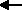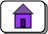created Jan 2, 1997; revised Dec 13, 2008, July 07, 2015

The binary addition algorithm operates on two bit patterns and results in a bit pattern. Usually all three patterns are the same size, and all three represent unsigned integers or all three represent signed integers.

Addition of one-bit binary operands is easy:

```       0    0    1    1
+0   +1   +0   +1
---  ---  ---  ---
00   01   01   10
```

These sums show one-bit operands and two-bit results. For multi-bit operands, the above sums are used for each column. The left-most bit of the one-bit result is used for the carry into the next column. For example, here is the sum of two four-bit integers:

```     0 1 1 0the carry into each column
0 1 1 0first operand
0 1 1 1second operand
-------
1 1 0 1the result
```

Adding the bits in one column produces a carry bit that is placed at the top of the next column to the left. This is called the carry out for this column and the carry in for next column left. Every column but the right-most includes a carry bit that comes from the column to its right. (Think of the right-most column as having a carry-in of zero.) Now columns have three bits to be added, and the addition rules must be extended:

```       0    0    0    0    1    1    1    1
0    0    1    1    0    0    1    1
+0   +1   +0   +1   +0   +1   +0   +1
---  ---  ---  ---  ---  ---  ---  ---
00   01   01   10   01   10   10   11
```

Of course, you don't have to memorize these rules. Just count the number of 1's in each column and write that count in binary.

Computers (usually) add two N-bit integers together to produce an N-bit result and a carry-out of the left-most column. Every bit of the result must have a value. The following shows an 8-bit addition:

```        0 0 0 0 1 1 0 0
0 0 0 0 1 1 1 0
0 0 0 0 0 1 0 1
---------------
0 0 0 1 0 0 1 1
```

The result is an N-bit pattern and a carry bit. The carry out from the left-most column might be zero or one. Each input pattern can be any pattern at all, and the algorithm will always produce an output pattern. However if the inputs are regarded as positive integers, some output patterns don't correspond to a correct sum. This is called overflow. Computer hardware looks at the carry into and out of the left-most column to determine if overflow happened.

Here is an implementation of this algorithm for 8-bit operands. Click on the bits of the operands X and Y to toggle the bits from 0 to 1 and back.

 Carry: X: Y: Sum:

Puzzles:

Find two non-zero operands that add up to a result of all one bits.

Find two non-zero operands that add up to a result of all zero bits.Return to Home Page